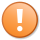Effective August 7, 2014Negative Boost Effects On The Opposite Element Were Removed

## Calculating Earnings Summary

The boosted earning rate can be calculated using the below equation:

Boosted Income = (Base Income x (1 + 0.3 * Number of boosts))

When multiplying if there is a decimal in your product round it up the next whole number.

Example: 202.27 would become 203
Number of
Positive Boosts
Multiply The Earning Rate
Without Boosts By
0 1.00
1 1.30
2 1.60
3 1.90
4 2.20

## Calculating Earnings With Positive Element Boosts

Boosts each provide an increase of 30% to the earning rates of dragons of the same element that are housed on the island that the boost is placed on. Depending on the boost and dragon placement, a dragon's earnings rates can be affected in a positive way.

Please refer to the Boosts page, as well as each boost's individual page, for more details.

Boosts do not affect epic dragons without primary elements (besides monolith dragons or any of the gemstone dragons at this time.

To calculate the earning rate of a dragon, the player will need to determine how many boosts are affecting that dragon. Then, the player will need to add that amount from his/her dragons' earnings. The effects of one positive boost on a dragon with a base earning rate of 100 would be calculated as:

100 + (100*30%) = 130

If a dragon's base earning rate is 100, and was then affected by two positive boosts, the calculation would be:

100 + 2*(100*30%) = 160

If a dragon's base earning rate is 100, and was then affected by three positive boosts, the calculation would be:

100 + 3*(100*30%) = 190

If a dragon's base earning rate is 100, and was then affected by four positive boosts, the calculation would be:

100 + 4*(100*30%) = 220

The generic formula to follow is:

The player simply needs to place the appropriate number of positive boosts into the formula for their given situation.

Boosted Income = (Base Income x (1 + 0.3 * Number of boosts))

• Note, multiplying a number by 1.3 is the same as adding on 30%.

When multiplying if there is a decimal in your product round it up the next whole number.

• Example: 202.27 would become 203

## Rounding Ranges

Once a dragon's displayed earn rate goes over 900 DragonCash per minute, a dragon's earn rate will get rounded up to cerain values depending on how much they earn.

Range of Displayed Rates Rounded To
900-999 1,000
1000-1,199 1,200
1,200-1,499 1,500
1,500-1,999 2,000
2,000-2,999 3,000

Example: A Tien Dragon's base earning rate at level 20 is 992, if affected by four positive boosts, the calculation would be:

992 * 4*(100*30%) = 2,182, which gets rounded up to 3,000.

## Dragon Earning Rates Tables

The following page has tables of the earning rate per minute each dragon earns without boosts. Use this page to apply your Boost Calculations to: